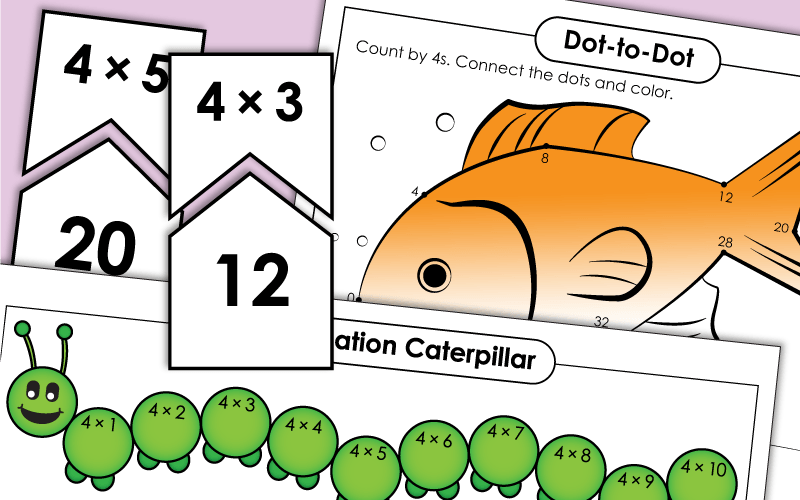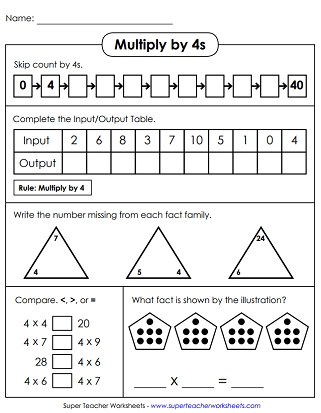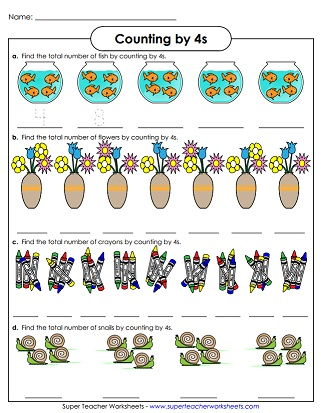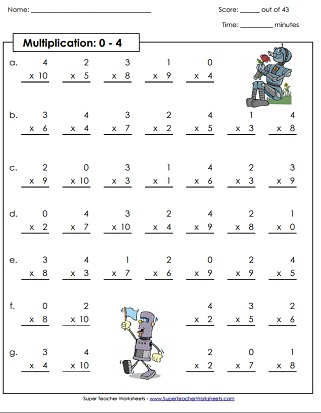# Multiplication by 4s

This page has a collection of worksheets for teaching kids to multiply by the number four.## Multiplication by4s Only

At the top of the worksheet, kids will skip count by 4s from 0 to 40. Then they'll complete the input-output boxes. After that they'll complete the fact family triangles.
Multiply numbers by 4 and write the answers. Then color each section of the picture the correct color according to the key. The finished product is an airplane.
In this file, you'll find 30 task cards. They have arrays, fact triangles, group models, and repeated addition expressions.
Students will cut apart all of these pieces and arrange them so the multiplication facts match the correct answer.
Multiply by 4s to in order to fill in all the bubbles on the multiplication caterpillar.
A cootie catcher is an origami toy. This one can be used to practice basic multiplication facts with factors of 4.
Print these flash cards and cut them apart for practice. There's also a sorting mat and a practice quiz.
Print this file on this card stock. Cut and color. Then use this dog slider craft to practice basic multiplication by 4.

## Skip Counting by 4s

Count the snails, crayons, flowers and fish. Objects are in groups of 4.
Skip count by 4s and write the numbers on the number line. Numbers up to 36 (3x12).
Count by 4s, from 0 to 40. Connect the dots to make a fish. Then answer several multiplication facts at the bottom of the page.
Write the missing numbers in the boxes. This worksheet has a skiing theme. Numbers up to 100.

## All Facts0s through 4s

This activity has 43 facts to practice multiplication facts with factors of 0, 1, 2, 3, and 4. Facts through 10, up to 4x10.
This sheet has about 40 facts to check student understanding of multiplication facts with factors of 0, 1, 2, 3, and 4. Facts through 12, up to 4x12.
This timed test covers all basic facts for 0s, 1s, 2s, 3s, and 4s. Facts through 12, up to 4x12.
Multiplication Basic Facts: 0-10

On this page you can find dozens of worksheets and games covering all basic facts up to 10x10 and 12x12.

Multiplication/Division
Fact Families

Teach students about the relationship between multiplication and division with these fact family worksheets.

Multiplication by 3s

This is a set of worksheets that specifically targets 3s times tables.

Multiplication by 5s

Here are the activities for learning multiplication facts from 5x0 up to 5x12.

Multiplication by 6s

Multiplication by 7s

Basic facts with factors of 7 are the focus on these printable learning resources.

Multiplication by 8s

Practice multiplying by the number 8. Includes mystery pictures, skip counting lessons, and more.

Multiplication by 9s

## Worksheet ImagesMy Account
Site Information